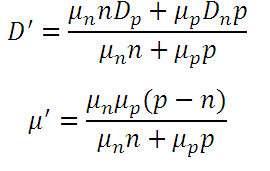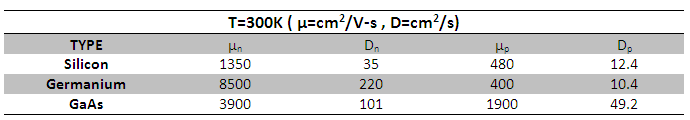# Ambipolar Transport Coefficients Calculator

This CalcTown calculator calculates the ambipolar transportation coefficients namely the ambipolar Diffusion coefficient D and mobility coefficient.

*Note that since D and µ are related to each other through Einstein's relation, only one of the two values are actually required to calculate the ambipolar transportation coefficients.

** Please use the calculator given in the first link in the section of related calculators to find the values of n and p for the given doping concentration.

cm2/V-s
cm2/V-s
cm-3
cm-3

#### Result

cm2/V-s
cm2/s